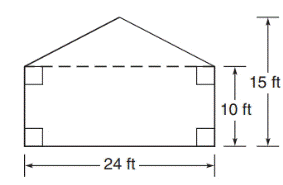Chapter 8.1, Problem 25E### Elementary Geometry for College St...

6th Edition
Daniel C. Alexander + 1 other
ISBN: 9781285195698

#### Solutions

Chapter
Section### Elementary Geometry for College St...

6th Edition
Daniel C. Alexander + 1 other
ISBN: 9781285195698
Textbook Problem
1 views

# The exterior wall (the gabled end of the house shown) remains to be painted.a) What is the area of the outside wall?b) If each gallon of paint covers approximately 105  ft 2 , how many gallons of paint must be purchased?c) If each gallon of paint is on sale for $22.50, what is the total cost of the paint?To determine a) The area of the outside wall. Explanation 1) Procedure: Finding the area of the figure is followed by identifying the figure and find area with the suitable geometry formulae. 2) Calculation: Given, In a given figure, the wall is a compound figure of triangle and rectangle. Area of the wall A=(A1) Area of a rectangular wall+(A2)Area of a triangular wall Where, Length of rectangle l=24 ft Width of rectangle w=10 ft Base of the triangle b=24 ft Height of the triangle h=(1510) ft h=5 ft. Area of a rectangular wall (A1)=lw unit2 To determine b) The gallons of paint must be purchased if each gallon of paint covers 105 ft2. To determine c) The total cost of paint, if each gallon of paint is on sale for$22.50

### Still sussing out bartleby?

Check out a sample textbook solution.

See a sample solution

#### The Solution to Your Study Problems

Bartleby provides explanations to thousands of textbook problems written by our experts, many with advanced degrees!

Get Started

#### Find for the functions in Problems 7-10. 10.

Mathematical Applications for the Management, Life, and Social Sciences

#### In Exercises 2340, find the indicated limit. 33. limx22x+1x+2

Applied Calculus for the Managerial, Life, and Social Sciences: A Brief Approach

#### By comparing areas, show that 12+13++1nlnn1+12+13++1n1

Single Variable Calculus: Early Transcendentals, Volume I

#### 2 1 0 does not exist

Study Guide for Stewart's Single Variable Calculus: Early Transcendentals, 8th